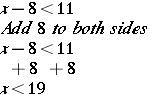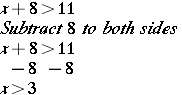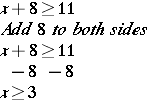# Solve Inequalities by Adding or Subtracting

In this lesson, you will use your equation skills as a basis for learning how to solve inequalities. You will see that the process for addition and subtraction of inequalities is the same as for equations as you continue to use inverse operations to solve them. There is one big difference in the outcome of an equation and an inequality. In an equation, you find the solution and in an inequality, you will find a solution set.

When you solve inequalities, you will use one of the following symbols:

Symbol Represents
< less than
> greater than
less than or equal to
greater than or equal to

To solve an inequality, you follow the same rules as when solving an equation. The solution of an inequality is a set of numbers known as the solution set. When you solve an equation, you check your work by substituting the number for the variable and solving the problem. When you solve an inequality, you select any number in the solution set and substitute that number for the variable.### Example 1

What is the solution set for this inequality?x can be ANY number less than 19, so the solution set is infinite.

Check: Select a number less than 19 and substitute for x.

10 < 19 Correct!### Example 2x can be ANY number greater than 3, so the solution set is infinite.

Check: Select a number greater than 3 and substitute for x.

5 > 3 Correct!

### Example 3

When you use these symbols: (≤, ≥) your solution set contains the number used to solve the inequality. If I replaced the symbol in example 2 with this symbol, 18 would be part of the solution set.x can be ANY number greater than 3, so the solution set is infinite.

Check: Select a number 3 or greater and substitute for x.

18 ≥ 3 Correct!## Investigate

Monday’s high temperature was 20°F. Tuesday’s high temperature was forecast to be no more than 8°F warmer than Monday’s high temperature. According to the forecast, what are the possible high temperatures for Tuesday?

Strategy

Now is the time to translate the words to math, using key words.

The words “no more than” indicate 8.

The word “was” indicates where to place the inequality sign.

Since Tuesday’s temps could be higher, subtract 20 from Tuesday’s temp (t).

t – 20 ≤ 8

Solution

The inequality t – 20 ≤ 8 represents the possible high temperatures for Tuesday. Once you have written the inequality, use inverse operations and solve, just as you would solve a simple equationThis means the possible high temperatures for Tuesday are 28°F or less.## Investigate

Let’s try another type of word problem!

The Y Club is sponsoring a trip to the Fox Theatre for a performance. However, there must be at least 130 members who will sign up or they cannot pay for the buses. So far, 98 members have signed up for the trip.  How many more members must sign up to make this trip possible?

Strategy

First, translate the words to math, using key words and phrases.

The words “at least 130” indicate ≥ 130.

The words “how many more” indicate addition.

You will need to combine (or add) the total members (m).

m + 98 ≤ 130

Solution

m + 98 ≤ 130
Subtract 98 from both sides of the inequality
m + 98 ≤ 130
– 98     – 98
m ≤ 32

This means that at least 32 Y Club members must sign up in order for the trip to be possible.

## Practice

1. a + 7 > 2
2. a – 9 ≤ 3
3. a – 8 < -16
4. 14 + a > -7
5. a – 17 ≥ -8
6. a + 25 ≤ 9
7.  a + 23 < -35
8. 54 + a ≤ 45

(source)